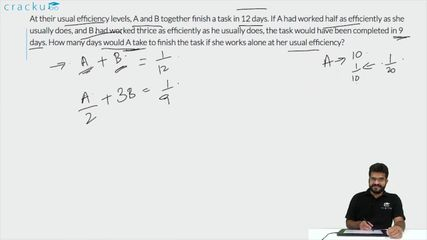Question 86

# At their usual efficiency levels, A and B together finish a task in 12 days. If A had worked half as efficiently as she usually does, and B had worked thrice as efficiently as he usually does, the task would have been completed in 9 days. How many days would A take to finish the task if she works alone at her usual efficiency?

Solution

Assuming A completes a units of work in a day and B completes B units of work in a day and the total work = 1 unit

Hence, 12(a+b)=1.........(1)

Also, 9($$\ \frac{\ a}{2}$$+3b)=1  .........(2)

Using both equations, we get, 12(a+b)= 9($$\ \frac{\ a}{2}$$+3b)

=> 4a+4b=$$\ \frac{\ 3a}{2}$$+9b

=> $$\ \frac{\ 5a}{2}$$=5b

=> a=2b

Substituting the value of b in equation (1),

12($$\ \frac{\ 3a}{2}$$)=1

=> a=$$\ \frac{\ 1}{18}$$

Hence, the number of days required = 1/($$\ \frac{\ 1}{18}$$)=18

### View Video Solution